# 深度学习基础：为什么神经网络的感知机中的神经元需要偏置项？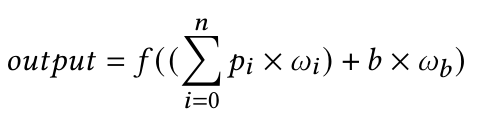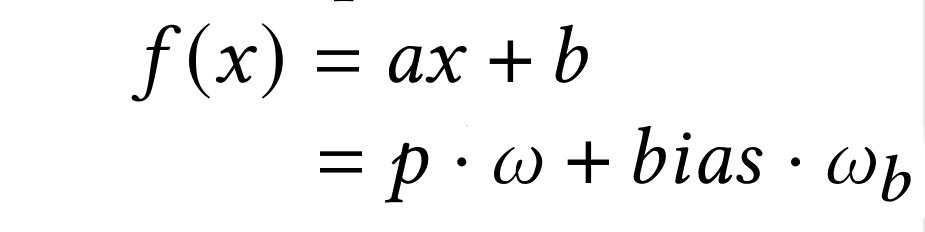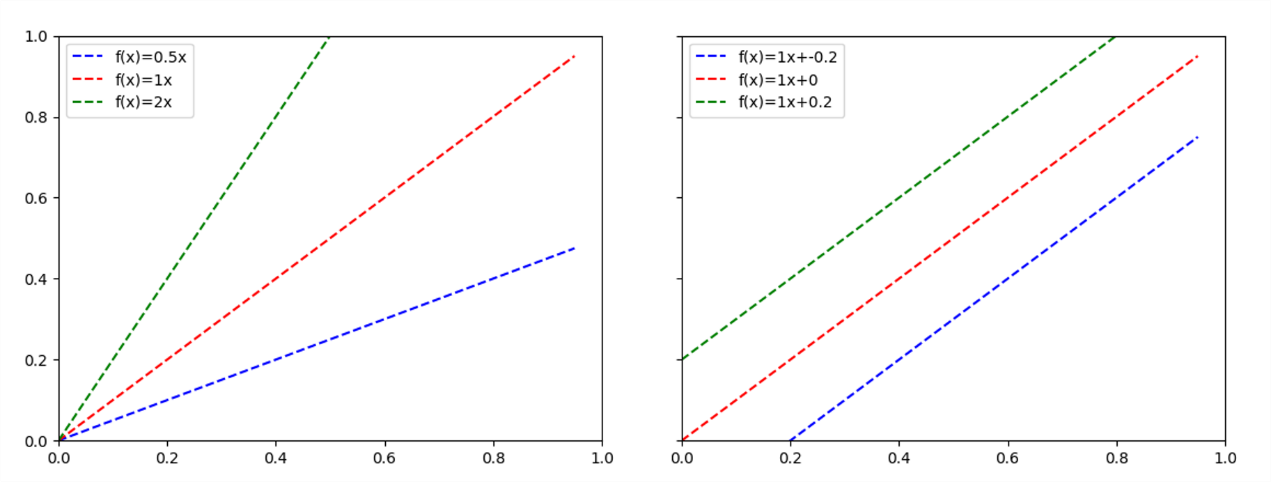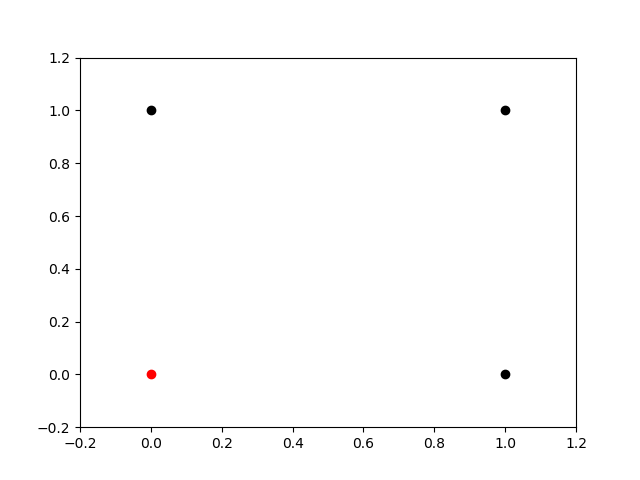classPerceptron():def__init__(self, n_input, alpha=0.01, has_bias=True):
self.has_bias = has_bias
self.bias_weight = random.uniform(-1,1)
self.alpha = alpha
self.weights = []
for i in range(n_input):
self.weights.append(random.uniform(-1,1))defclassify(self, input):
summation = 0if(self.has_bias):
summation += self.bias_weight * 1for i in range(len(self.weights)):
summation += self.weights[i] * input[i]
return self.activation(summation)defactivation(self, value):if(value < 0):
return0else:
return1deftrain(self, input, target):
guess = self.classify(input)
error = target - guess
if(self.has_bias):
self.bias_weight += 1 * error * self.alpha
for i in range(len(self.weights)):
self.weights[i] += input[i] * error * self.alpha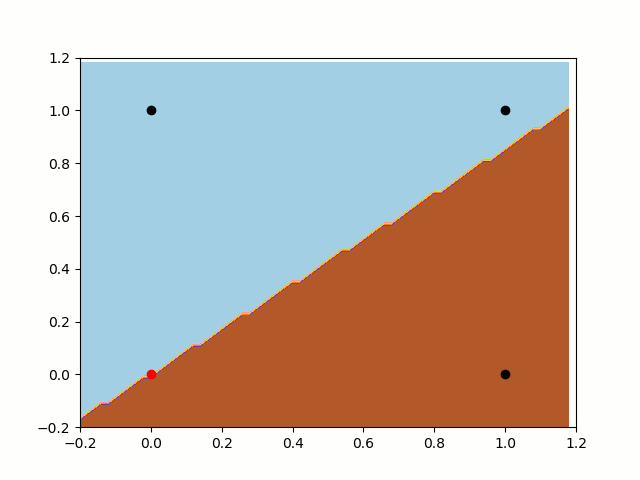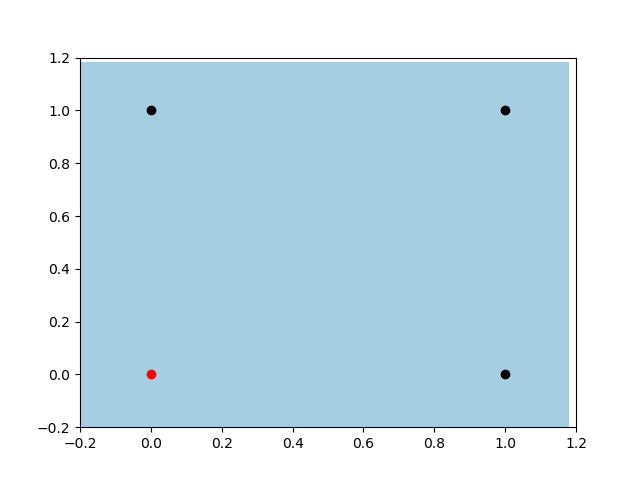deephub翻译组：zhangzc08-23375512-315455
09-061万+
06-1715万+
05-312797
12-165万+
02-20592
09-15123
10-11115
06-183682
01-231319
03-05513
08-022964
11-18847
05-01146
09-23110
11-02344
01-20713